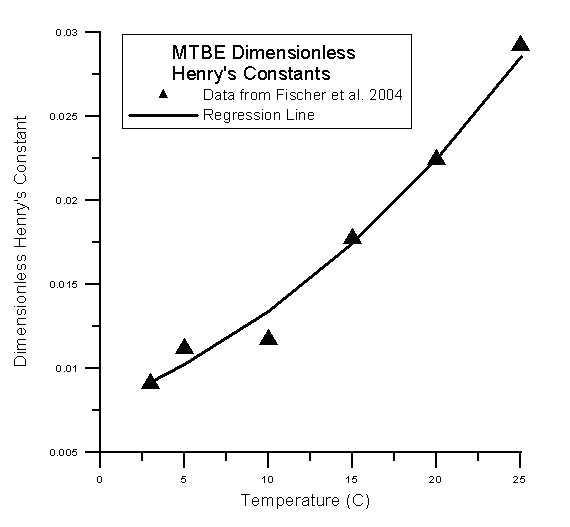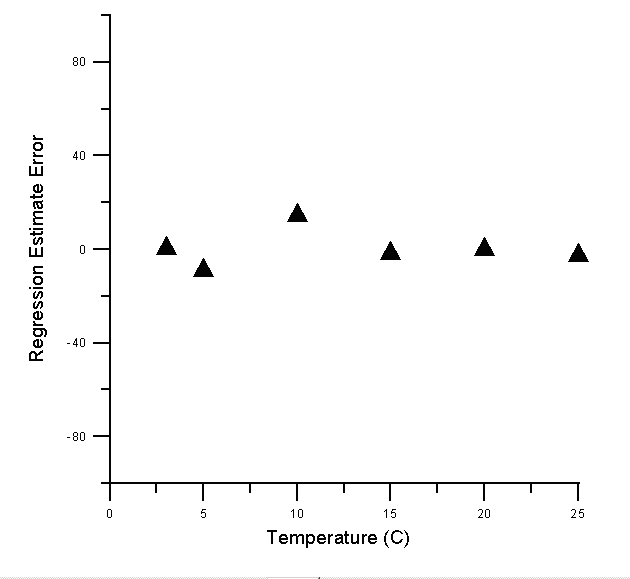# EPA On-line Tools for Site Assessment Calculation

 39 of 67

Data for MTBE were selected from Fischer et al. (2004)1. These data were used to approximate the van't Hoff relationship for MTBE by fitting a linear regression through the ln Kh versus the inverse temperature, TK in Kelvin (273 K = 0 oC), plot. The regression equation was ln Kh = -4256.2 (1/TK) + 6.927 for Kh in units of atm - m3 / mol. As a measure of the fit of the equation to the data, the R2 was 0.9729.

After fitting the relationship, the original data (triangles) were plotted against the estimates (curve). (The estimates follow a curve, because the linear regression was taken in the log space.)In absolute terms, the errors in the estimates were found to range from 0 to 14.5% as shown in the following table and graph.

Temperature oC % Error in Henry's Constant Estimate
3 0.549451
5 -8.9285
10 14.5299
15 -1.69492
20 0
25 -2.39726#### References

1Fischer, A., M. Muller, J. Klasmeier, 2004, Determination of Henry's law constant for mthyl tert-butyl ether (MTBE) at groundwater temperatures, Chemosphere, 54, 689-694.

 Top ^ Home | Glossary | Notation | Links | References | Calculators

Contact Jim Weaver to ask a technical question on this material.